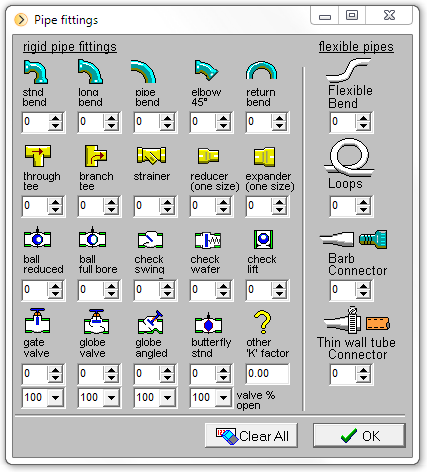# Pipe Fittings DatabasePipe Flow Wizard comes with its own Pipe Fittings Database that contains K factors for many different types and sizes of pipe bends, valves, contractions and expansions. A fitting's K factor allows the pressure drop across the fitting to be calculated for a specific velocity of fluid flow through the pipe fitting. i.e the pressure drop through the fitting varies depending on the fluid velocity and use of an associated K factor allows the fitting pressure loss to be calculated for a particular flow rate.

## Pressure Loss through a Pipe Fitting

The pressure loss through a fitting (m hd or ft.hd) is calculated using the 'K' factor as:

Fitting Head Loss = Kv² / 2g

where:

K= K factor associated with a specific type and size of fitting
v = fluid velocity (m/s or ft/s)
g = 9.806 m/s² or 32.174 ft/s²

## Pipe Fittings Database

Within the Pipe Flow Wizard software the type and quantity of bends, valves and other fittings associated with the pipe can be set by amending the quantity values on the 'Pipe fittings' screen.• Click on the 'Valve' button to display the 'Pipe fittings' screen.
• Enter the quantity of bends, fittings, valves etc. in the appropriate input boxes.

The total quantity of fittings is displayed on the 'Valve' button on the calculation screen.

## Bespoke Pipe Fittings and Components

Bespoke fittings can be modelled by using the "Other K factor" input box, where an K factor can be entered manually. This allows the user to include the pressure drop of any fitting in the calculation.

Where two or more bespoke fittings are to be modelled, the 'K' factor for each fitting should be summed and the total 'K' value should be entered.

Note: When performing a 'Find diameter' calculation, it may be necessary to first estimate the pipe diameter without any fitting data, in order to allow the 'K' factor to be established for any bespoke fittings.

## Pipe Fittings - Minor Losses

In many systems, the effect of pipe fittings are considered as minor losses, since the friction losses in the pipes are often significantly higher than the pressure loss through the fittings. This is not always the case but for systems with a reasonable length of pipe and not too many fittings, it is fairly common.

## Equivalent Length of Pipe for Pipe Fittings

Sometimes the pressure loss of a fitting is expressed as an 'Equivalent length' of pipe, where by the engineer calculates a further length of pipe that will produce an extra friction loss in the pipe that is equivalent to the loss through the fitting. In this way, adding a notional extra length to each pipe can model the further pressure loss that would have occured due to the fittings.

However, the Pipe Flow Wizard software does not allow the use of equivalent length of pipe. It actually calculates the fittings pressure loss using well known and established fitting loss equations.

The 'K' factor of a fitting may be calculated from the 'Equivalent length' (in m or ft.) if the friction factor and the Internal diameter (in m or ft.) are known.

The 'Equivalent length' and 'Internal diameter' must be in the same units to calculate the 'K' factor.

K = (EL * ff) / i.d.

where:

EL= Equivalent length of pipe (in m or ft)
ff = Friction factor
i.d. = Internal Diameter of the pipe (in m or ft, same as for EL)

Next: Pipe Flow Wizard Fluids Database

Secure Online PaymentsAnd
Like Pipe Flow here:Pipe Flow Software: Piping design, Pressure drop calculator, Flow rate calculator, Pump head calculations, Pump selection software.  Copyright © Pipe Flow Software 1997-2020